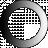# American Dictionary of the English Language

Dictionary Search

### Square

1. Having four equal sides and four right angles; as a square room; a square figure.

2. Forming a right angle; as an instrument for striking lines square

3. Parallel; exactly suitable; true.

Shes a most triumphant lady, if report be square to her. [Unusual.]

4. Having a straight front, or a frame formed with straight lines; not curving; as a man of a square frame; a square built man.

5. That does equal justice; exact; fair; honest; as square dealing.

6. Even; leaving no balance. Let us make or leave the accounts square

Three square five square having three or five equal sides, etc.; an abusive use of square

SQUARE root, in geometry and arithmetic. The square root of a quantity or number is that which, multiplied by itself, produces the square Thus 7 is the square root of 49, for 7 times 7 = 49.

In seamens language, the yards are square when they are arranged at right angles with the mast or the keel. The yards and sails are said also to be square when they are of greater extent than usual.

SQUARE, noun

1. A figure having four equal sides and four right angles.

2. An area of four sides, with houses on each side.

The statue of Alexander VII. stands in the large square of the town.

3. The content of the side of a figure squared.

4. An instrument among mechanics by which they form right angles, or otherwise measure angles.

5. Im geometry and arithmetic, a square or square number is the product of a number multiplied by itself. Thus 64 is the square of 8, for 8 times 8 = 64.

6. Rule; regularity; exact proportion; justness of workmanship and conduct.

They of Galatia much more out of square

I have not kept my square [Not in use.]

7. A square body of troops; a squadron; as the brave square of war. [Not in use.]

8. A quaternion; four. [Not in use.]

9. Level; equality.

We live not on the square with such as these.

10. In astrology, quartile; the position of planets distant ninety degrees from each other.

11. Rule; conformity; accord. I shall break no squares with another for a trifle.

SQUAREs go. Let us see how the squares go, that is, how the game proceeds; a phrase taken from the game of chess, the chess board being formed with squares.

SQUARE, verb transitive

1. To form with four equal sides and four right angles.

2. To reduce to a square; to form to right angles; as, to square masons work.

3. To reduce to any given measure or standard.

4, To adjust; to regulate; to mold; to shape; as, to square our actions by the opinions of others; to square our lives by the precepts of the gospel.

5. To accommodate; to fit; as, square my trial to my strength.

6. To respect in quartile.

7. To make even, so as to leave no difference or balance; as, to square accounts; a popular phrase.

8. In arithmetic, to multiply a number by itself; as, to square the number.

9. In seamens language, to square the yards, is to place them at right angles with the mast or keel.

SQUARE, verb intransitive

1. To suit; to fit; to quadrate; to accord or agree. His opinions do not square with the doctrines of philosophers.

2. To quarrel; to go to opposite sides.

Are you such fools to square for this? [Not in use.]